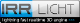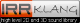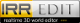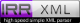Retour
Version Originale

#### ./aip/1.8aipmod/include/plane3d.h :


// Copyright (C) 2002-2011 Nikolaus Gebhardt

// This file is part of the "Irrlicht Engine".

// For conditions of distribution and use, see copyright notice in irrlicht.h

#ifndef __IRR_PLANE_3D_H_INCLUDED__
#define __IRR_PLANE_3D_H_INCLUDED__

#include "irrMath.h"
#include "vector3d.h"

namespace irr
{
namespace core
{

//! Enumeration for intersection relations of 3d objects

enum EIntersectionRelation3D
{
ISREL3D_FRONT = 0,
ISREL3D_BACK,
ISREL3D_PLANAR,
ISREL3D_SPANNING,
ISREL3D_CLIPPED
};

//! Template plane class with some intersection testing methods.

template <class T>
class plane3d
{
public:

// Constructors

plane3d(): Normal(0,1,0) { recalculateD(vector3d<T>(0,0,0)); }

plane3d(const vector3d<T>& MPoint, const vector3d<T>& Normal) : Normal(Normal) { recalculateD(MPoint); }

plane3d(T px, T py, T pz, T nx, T ny, T nz) : Normal(nx, ny, nz) { recalculateD(vector3d<T>(px, py, pz)); }

plane3d(const vector3d<T>& point1, const vector3d<T>& point2, const vector3d<T>& point3)
{ setPlane(point1, point2, point3); }

plane3d(const vector3d<T> & normal, const T d) : Normal(normal), D(d) { }

// operators

inline bool operator==(const plane3d<T>& other) const { return (equals(D, other.D) && Normal==other.Normal);}

inline bool operator!=(const plane3d<T>& other) const { return !(*this == other);}

// functions

void setPlane(const vector3d<T>& point, const vector3d<T>& nvector)
{
Normal = nvector;
recalculateD(point);
}

void setPlane(const vector3d<T>& nvect, T d)
{
Normal = nvect;
D = d;
}

void setPlane(const vector3d<T>& point1, const vector3d<T>& point2, const vector3d<T>& point3)
{
// creates the plane from 3 memberpoints

Normal = (point2 - point1).crossProduct(point3 - point1);
Normal.normalize();

recalculateD(point1);
}

//! Get an intersection with a 3d line.

/** \param lineVect Vector of the line to intersect with.
\param linePoint Point of the line to intersect with.
\param outIntersection Place to store the intersection point, if there is one.
\return True if there was an intersection, false if there was not.
*/
bool getIntersectionWithLine(const vector3d<T>& linePoint,
const vector3d<T>& lineVect,
vector3d<T>& outIntersection) const
{
T t2 = Normal.dotProduct(lineVect);

if (t2 == 0)
return false;

T t =- (Normal.dotProduct(linePoint) + D) / t2;
outIntersection = linePoint + (lineVect * t);
return true;
}

//! Get percentage of line between two points where an intersection with this plane happens.

/** Only useful if known that there is an intersection.
\param linePoint1 Point1 of the line to intersect with.
\param linePoint2 Point2 of the line to intersect with.
\return Where on a line between two points an intersection with this plane happened.
For example, 0.5 is returned if the intersection happened exactly in the middle of the two points.
*/
f32 getKnownIntersectionWithLine(const vector3d<T>& linePoint1,
const vector3d<T>& linePoint2) const
{
vector3d<T> vect = linePoint2 - linePoint1;
T t2 = (f32)Normal.dotProduct(vect);
return (f32)-((Normal.dotProduct(linePoint1) + D) / t2);
}

//! Get an intersection with a 3d line, limited between two 3d points.

/** \param linePoint1 Point 1 of the line.
\param linePoint2 Point 2 of the line.
\param outIntersection Place to store the intersection point, if there is one.
\return True if there was an intersection, false if there was not.
*/
bool getIntersectionWithLimitedLine(
const vector3d<T>& linePoint1,
const vector3d<T>& linePoint2,
vector3d<T>& outIntersection) const
{
return (getIntersectionWithLine(linePoint1, linePoint2 - linePoint1, outIntersection) &&
outIntersection.isBetweenPoints(linePoint1, linePoint2));
}

//! Classifies the relation of a point to this plane.

/** \param point Point to classify its relation.
\return ISREL3D_FRONT if the point is in front of the plane,
ISREL3D_BACK if the point is behind of the plane, and
ISREL3D_PLANAR if the point is within the plane. */
EIntersectionRelation3D classifyPointRelation(const vector3d<T>& point) const
{
const T d = Normal.dotProduct(point) + D;

if (d < -ROUNDING_ERROR_f32)
return ISREL3D_BACK;

if (d > ROUNDING_ERROR_f32)
return ISREL3D_FRONT;

return ISREL3D_PLANAR;
}

//! Recalculates the distance from origin by applying a new member point to the plane.

void recalculateD(const vector3d<T>& MPoint)
{
D = - MPoint.dotProduct(Normal);
}

//! Gets a member point of the plane.

vector3d<T> getMemberPoint() const
{
return Normal * -D;
}

//! Tests if there is an intersection with the other plane

/** \return True if there is a intersection. */
bool existsIntersection(const plane3d<T>& other) const
{
vector3d<T> cross = other.Normal.crossProduct(Normal);
return cross.getLength() > core::ROUNDING_ERROR_f32;
}

//! Intersects this plane with another.

/** \param other Other plane to intersect with.
\param outLinePoint Base point of intersection line.
\param outLineVect Vector of intersection.
\return True if there is a intersection, false if not. */
bool getIntersectionWithPlane(const plane3d<T>& other,
vector3d<T>& outLinePoint,
vector3d<T>& outLineVect) const
{
const T fn00 = Normal.getLength();
const T fn01 = Normal.dotProduct(other.Normal);
const T fn11 = other.Normal.getLength();
const f64 det = fn00*fn11 - fn01*fn01;

if (fabs(det) < ROUNDING_ERROR_f64 )
return false;

const f64 invdet = 1.0 / det;
const f64 fc0 = (fn11*-D + fn01*other.D) * invdet;
const f64 fc1 = (fn00*-other.D + fn01*D) * invdet;

outLineVect = Normal.crossProduct(other.Normal);
outLinePoint = Normal*(T)fc0 + other.Normal*(T)fc1;
return true;
}

//! Get the intersection point with two other planes if there is one.

bool getIntersectionWithPlanes(const plane3d<T>& o1,
const plane3d<T>& o2, vector3d<T>& outPoint) const
{
vector3d<T> linePoint, lineVect;
if (getIntersectionWithPlane(o1, linePoint, lineVect))
return o2.getIntersectionWithLine(linePoint, lineVect, outPoint);

return false;
}

//! Test if the triangle would be front or backfacing from any point.

/** Thus, this method assumes a camera position from
which the triangle is definitely visible when looking into
the given direction.
Note that this only works if the normal is Normalized.
Do not use this method with points as it will give wrong results!
\param lookDirection: Look direction.
\return True if the plane is front facing and
false if it is backfacing. */
bool isFrontFacing(const vector3d<T>& lookDirection) const
{
const f32 d = Normal.dotProduct(lookDirection);
return F32_LOWER_EQUAL_0 ( d );
}

//! Get the distance to a point.

/** Note that this only works if the normal is normalized. */
T getDistanceTo(const vector3d<T>& point) const
{
return point.dotProduct(Normal) + D;
}

//! Normal vector of the plane.

vector3d<T> Normal;

//! Distance from origin.

T D;
};

//! Typedef for a f32 3d plane.

typedef plane3d<f32> plane3df;

//! Typedef for an integer 3d plane.

typedef plane3d<s32> plane3di;

} // end namespace core

} // end namespace irr

#endif


Correctionsxhtml 1.0
css 2.1
Propulsé par FluxBB